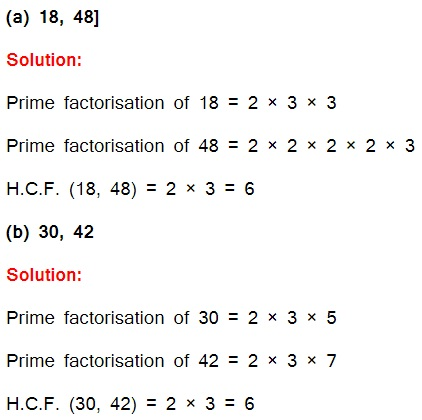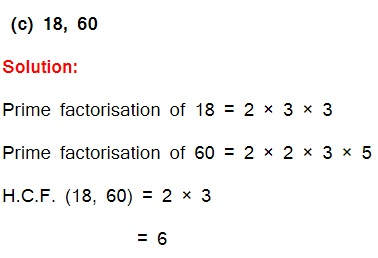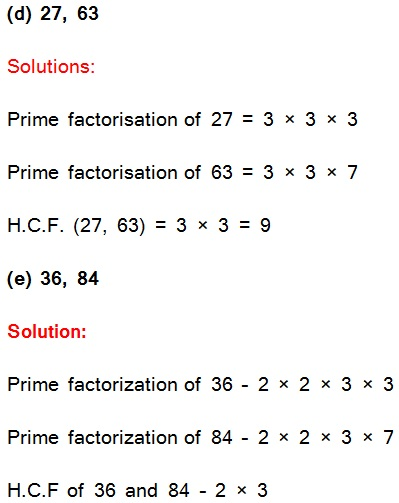Study Materials: ncert solutions

Our ncert solutions for Exercise 3.6 Class 6 maths 3. Playing with Numbers - ncert solutions - Toppers Study is the best material for English Medium students cbse board and other state boards students.

Solutions ⇒ Class 6th ⇒ Mathematics ⇒ 3. Playing with Numbers

# Exercise 3.6 Class 6 maths 3. Playing with Numbers - ncert solutions - Toppers Study

Topper Study classes prepares ncert solutions on practical base problems and comes out with the best result that helps the students and teachers as well as tutors and so many ecademic coaching classes that they need in practical life. Our ncert solutions for Exercise 3.6 Class 6 maths 3. Playing with Numbers - ncert solutions - Toppers Study is the best material for English Medium students cbse board and other state boards students.

## Exercise 3.6 Class 6 maths 3. Playing with Numbers - ncert solutions - Toppers Study

CBSE board students who preparing for class 6 ncert solutions maths and Mathematics solved exercise chapter 3. Playing with Numbers available and this helps in upcoming exams 2023-2024.

### You can Find Mathematics solution Class 6 Chapter 3. Playing with Numbers

• All Chapter review quick revision notes for chapter 3. Playing with Numbers Class 6
• NCERT Solutions And Textual questions Answers Class 6 Mathematics
• Extra NCERT Book questions Answers Class 6 Mathematics
• Importatnt key points with additional Assignment and questions bank solved.

NCERT Solutions do not only help you to cover your syllabus but also will give to textual support in exams 2023-2024 to complete exercise 3.6 maths class 6 chapter 3 in english medium. So revise and practice these all cbse study materials like class 6 maths chapter 3.6 in english ncert book. Also ensure to repractice all syllabus within time or before board exams for ncert class 6 maths ex 3.6 in english.

See all solutions for class 6 maths chapter 3 exercise 3 in english medium solved questions with answers.

### Exercise 3.6 class 6 Mathematics Chapter 3. Playing with Numbers

• Exercise 3.6 Class 6 Maths 3. Playing With Numbers - Ncert Solutions - Toppers Study
• Class 6 Ncert Solutions
• Solution Chapter 3. Playing With Numbers Class 6
• Solutions Class 6
• Chapter 3. Playing With Numbers Exercise 3.6 Class 6

## Exercise 3.6 Class 6 maths 3. Playing with Numbers - ncert solutions - Toppers Study

Exercise -3.6

Q1. Find the H.C.F. of the following numbers:(f) 34, 102

Solutions:

Prime factorization of 34

= 2 × 17

Prime factorization of 102

= 2 × 3 × 17

HCF of 34 and 102 is 2 × 17

= 34

(g) 70, 105, 175

Solution:

Prime factorization of 70

= 2 × 5 × 7

Prime factorization of 105

= 3 × 5 × 7

Prime factorization of 175

= 5 × 5 × 7

H.C.F. (70, 105, 175)

= 5 × 7

= 35

(h) 91, 112, 49

Solution:

Prime factorization of 91

= 7 × 13

Prime factorization of 112

= 2 × 2 × 2 × 2 × 7

Prime factorization of 49

= 7 × 7

H.C.F. (91, 112, 49) = 7

(i) 18, 54, 81

Solution:

Prime factorization of 18

= 2 × 3 × 3

Prime factorization of 54

= 2 × 3 × 3 × 3

Prime factorization of 81

= 3 × 3 × 3 × 3

H.C.F. = 3 × 3 = 9

(j) 12, 45, 75

Solution:

Prime factorization of 12

= 2 × 2 × 3

Prime factorization of 45

= 3 × 3 × 5

Prime factorization of 75

= 3 x 5 x 5

H.C.F. = 1 × 3

= 3

Q2. What is the H.C.F. of two consecutive:

(a) numbers?

(b) even numbers?

(c) odd numbers?

Solution:

(a) H.C.F. of two consecutive numbers be 1.

(b) H.C.F. of two consecutive even numbers be 2.

(c) H.C.F. of two consecutive odd numbers be 1.

Q3. H.C.F. of co-prime numbers 4 and 15 was found as follows by factorization:

4 = 2 × 2 and

15 = 3 × 5 since there is no common prime factor, so H.C.F. of 4 and 15 is 0. Is the answer correct? If not, what is the correct H.C.F.?

Solution: No. The correct H.C.F. is 1.

Important Study materials for classes 06, 07, 08,09,10, 11 and 12. Like CBSE Notes, Notes for Science, Notes for maths, Notes for Social Science, Notes for Accountancy, Notes for Economics, Notes for political Science, Noes for History, Notes For Bussiness Study, Physical Educations, Sample Papers, Test Papers, Mock Test Papers, Support Materials and Books.Mathematics Class - 11th

NCERT Maths book for CBSE Students.

books

## Study Materials List:

##### Solutions ⇒ Class 6th ⇒ Mathematics
1. Knowing Our Numbers
2. Whole Numbers
3. Playing with Numbers
4. Basic Geometrical Ideas
5. Understanding Elementary Shapes
6. Integers
7. Fractions
8. Decimals

New Books